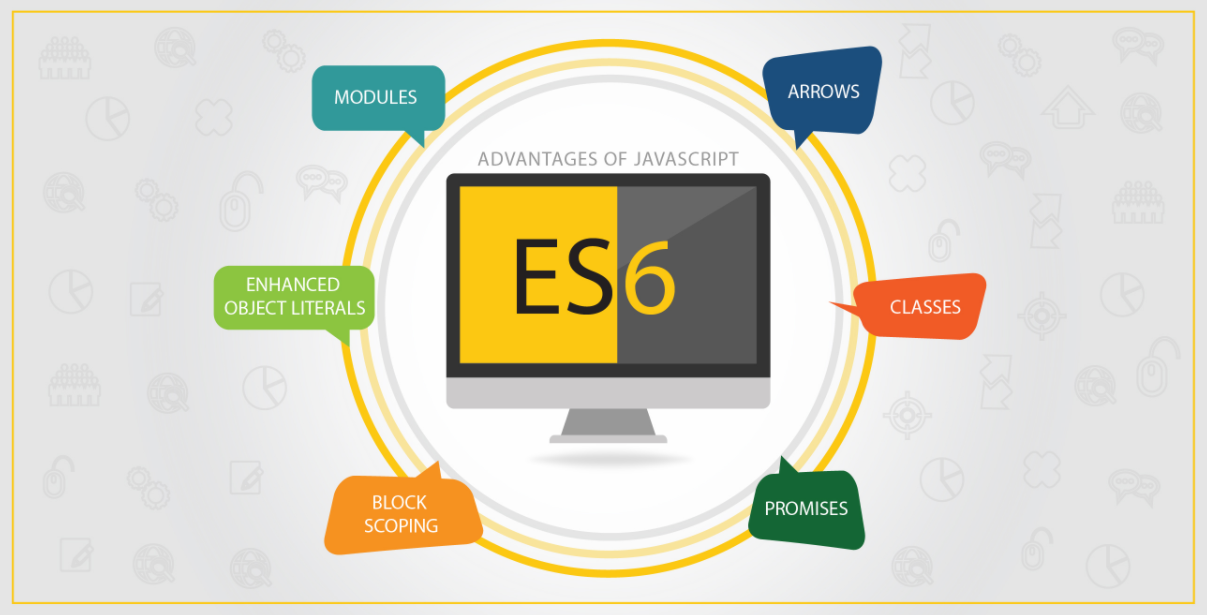## 1. JavaScript是一门面向对象的语言

``````//定义Person构造函数
function Person(name, id) {
this.name = name;
this.id = id;
}

//在Person.prototype中加入方法
Person.prototype.sayHi = function() {
console.log('你好, 我是' +  this.name);
}
``````

``````//实例化对象
let p1 = new Person('阿辉', 1234);

//调用sayHi方法
p1.sayHi();
``````

``````function Person(name, id) {
this.name = name;
this.id = id;
let salary = 20000;
this.getSalary = function (pwd) {
pwd === 123456 ? console.log(salary) : console.log('对不起, 你没有权限查看密码');
}
}
``````

``````function Student(name, id, subject) {
//使用call实现父类继承
Person.call(this, name, id);
//添加子类的属性
this.subject = subject;
}

let s1 = new Student('阿辉', 1234, '前端开发');
``````

JavaScript中函数没有重载， 所以JavaScript中的多态是靠函数覆盖实现的。

``````function Person(name, id) {
this.name = name;
this.id = id;
this.study = function() {
console.log(name + '在学习');
}
}
``````

``````function Student(subject) {
this.subject = subject;
this.study = function() {
console.log(this.name + '在学习' + this.subject);
}
}
Student.prototype = new Person('阿辉', 1234);
Student.prototype.constructor = Student;

function Teacher(subject) {
this.subject = subject;
this.study = function() {
console.log(this.name + '为了教学而学习' + this.subject);
}
}
Teacher.prototype = new Person("老夫子", 4567);
Teacher.prototype.constructor = Teacher;
``````

``````function doStudy(role) {
if(role instanceof Person) {
role.study();
}
}
``````

``````let student = new Student('前端开发');
let teacher = new Teacher('前端开发');

doStudy(student); //阿辉在学习前端开发
doStudy(teacher); //老夫子为了教学在学习前端开发
``````

JavaScript的面向对象

## 二. ES5中的面向对象

*这里的ES5并不特指ECMAScript 5， 而是代表ECMAScript 6 之前的ECMAScript！

## 1. 使用对象字面量的方式

``````var student1 = {
name: '阿辉',
age: 22,
subject: '前端开发'
};

var student2 = {
name: '阿傻',
age: 22,
subject: '大数据开发'
};
``````

• 在生成多个实例对象时， 我们需要每次重复写`name`,`age`,`subject`属性，写起来特别的麻烦
• 虽然都是学生的对象， 但是看不出`student1``student2`之间有什么联系。

## 2. 使用构造函数的方式

``````function Student (name, age, subject) {
this.name = name;
this.age = age;
this.subject = subject;
console.log(this);
}
``````

``````Student('阿辉', 22, '前端开发'); //window{}
``````

``````let student1 = new Student('阿辉', 22, '前端开发'); //Student {name: "阿辉", age: 22, subject: "前端开发"}

``````

``````let student1 = new Student('阿辉', 22, '前端开发');
let student2 = new Student('阿傻', 22, '大数据开发');
let student3 = new Student('阿呆', 22, 'Python');
let student4 = new Student('阿笨', 22, 'Java');
``````

## 1.`prototype`的原型继承

`prototype`是JavaScript这类基于原型继承的核心， 只要弄明白了原型和原型链， 就基本上完全理解了JavaScript中对象的继承。下面我将着重的讲解为什么要使用`prototype`和使用`prototype`实现继承的方式。

``````function Student (name, age, subject) {
this.name = name;
this.age = age;
this.subject = subject;
this.study = function() {
console.log('我在学习' + this.subject);
}
}
``````

``````let student1 = new Student('阿辉', 22, '前端开发');
let student2 = new Student('阿傻', 22, '大数据开发');

student1.study(); //我在学习前端开发
student2.study(); //我在学习大数据开发

``````

``````console.log(student1.study === student2.study); //false
``````

String中的方法

``````function Student (name, age, subject) {
this.name = name;
this.age = age;
this.subject = subject;
}
Student.prototype.study = function() {
console.log('我在学习' + this.subject);
}
``````

``````let student1 = new Student('阿辉', 22, '前端开发');
let student2 = new Student('阿傻', 22, '大数据开发');

student1.study(); //我在学习前端开发
student2.study(); //我在学习大数据开发

console.log(student1.study === student2.study); //true
``````

## 如何使用`prototype`实现继承？

“学生”这个对象可以分为小学生， 中学生和大学生等。我们现在新建一个小学生的构造函数`Pupil`

``````function Pupil(school) {
this.school = school;
}
``````

``````Pupil.prototype = new Student('小辉', 8, '小学义务教育课程');
Pupil.prototype.constructor = Pupil;

let pupil1 = new Pupil('北大附小');
``````

``````Pupil.prototype = new Student('小辉', 8, '小学义务教育课程');
``````

``````Pupil.prototype.constructor = Pupil;
``````

`Pupil`作为构造函数有一个`protoype`属性指向原型对象`Pupil.prototype`，而原型对象`Pupil.prototype`也有一个`constructor`属性指回它的构造函数`Pupil`。如下图所示：

prototype和constructor的指向

prototype和constructor的指向错误

``````Pupil.prototype = new Student('小辉', 8, '小学义务教育课程');
let pupil1 = new Pupil('北大附小');

console.log(pupil1.constructor === Student); //true
``````

``````Pupil.prototype = new Student('小辉', 8, '小学义务教育课程');

//修正constructor的指向错误
Pupil.prototype.constructor = Pupil;

let pupil1 = new Pupil('北大附小');

console.log(pupil1.constructor === Student); //false
console.log(pupil1.constructor === Pupil); //ture
``````

## 2. 使用`call`和`apply`方法实现继承

``````//父类构造函数
function Student (name, age, subject) {
this.name = name;
this.age = age;
this.subject = subject;
}

//子类构造函数
function Pupil(name, age, subject, school) {
//使用call实现继承
Student.call(this, name, age, subject);
this.school = school;
}

//实例化Pupil
let pupil2 = new Pupil('小辉', 8, '小学义务教育课程', '北大附小');
``````

``````//父类构造函数
function Student (name, age, subject) {
this.name = name;
this.age = age;
this.subject = subject;
}
//原型上挂载study方法
Student.prototype.study = function() {
console.log('我在学习' + this.subject);
}

//子类构造函数
function Pupil(name, age, subject, school) {
//使用call实现继承
Student.call(this, name, age, subject);
this.school = school;
}

let pupil2 = new Pupil('小辉', 8, '小学义务教育课程', '北大附小');

//报错
pupil2.study(); //Uncaught TypeError: pupil2.study is not a function
``````

``````//父类构造函数
function Student (name, age, subject) {
this.name = name;
this.age = age;
this.subject = subject;
}

//子类构造函数
function Pupil(name, age, subject, school) {
//使用applay实现继承
Student.apply(this, [name, age, subject]);
this.school = school;
}

//实例化Pupil
let pupil2 = new Pupil('小辉', 8, '小学义务教育课程', '北大附小');
``````

## 3. 其他继承方式

JavaScript中的继承方式不仅仅只有上面提到的几种方法， 在《JavaScript高级程序设计》中， 还有实例继承，拷贝继承，组合继承，寄生组合继承等众多继承方式。在寄生组合继承中， 就很好的弥补了`call``apply`无法继承原型属性和方法的缺陷，是最完美的继承方法。这里就不详细的展开论述，感兴趣的可以自行阅读《JavaScript高级程序设计》。

## (一) ES6中对象的创建

``````//定义类
class Student {
//构造方法
constructor(name, age, subject) {
this.name = name;
this.age = age;
this.subject = subject;
}

//类中的方法
study(){
console.log('我在学习' + this.subject);
}
}

//实例化类
let student3 = new Student('阿辉', 24, '前端开发');
student3.study(); //我在学习前端开发
``````

`Student`类除了构造函数方法，还定义了一个`study`方法。需要特别注意的是，在ES6中定义类中的方法的时候，前面不需要加上`function`关键字，直接把函数定义进去就可以了。另外，方法之间不要用逗号分隔，加了会报错。而且，类中的方法全部是定义在原型上的，我们可以用下面的代码进行验证。

``````console.log(student3.__proto__.study === Student.prototype.study); //true
console.log(student3.hasOwnProperty('study')); // false
``````

## (二) ES6中对象的继承

E6中`class`可以通过`extends`关键字来实现继承， 这比前面提到的ES5中使用原型链来实现继承， 要清晰和方便很多。下面我们使用ES6的语法来实现`Pupil`

``````//子类
class Pupil extends Student{
constructor(name, age, subject, school) {
//调用父类的constructor
super(name, age, subject);
this.school = school;
}
}

let pupil = new Pupil('小辉', 8, '小学义务教育课程', '北大附小');
pupil.study(); //我在学习小学义务教育课程
``````

## 四.结束语

JavaScript 被认为是世界上最受误解的编程语言，因为它身披 c 语言家族的外衣，表现的却是 LISP 风格的函数式语言特性；没有类，却实也彻底实现了面向对象。要对这门语言有透彻的理解，就必须扒开其 c 语言的外衣，从新回到函数式编程的角度，同时摒弃原有类的面向对象概念去学习领悟它(摘自参考目录1)。现在的前端中不仅普遍的使用了ES6的新语法，而且在JavaScript的基础上还出现了TypeScript、CoffeeScript这样的超集。可以预见的是，目前在前端生态圈一片繁荣的情况下，对JSer的需求也会越来越多，但同时也对前端开发者的JavaScript的水平提出了更加严苛的要求。使用面向对象的思想去开发前端项目也是未来对JSer的基本要求之一！